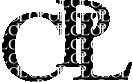Page: (graphics)Top, Next: nographics, Prev: (matrix), Up: (cpl)Library Index

### Plotting library

The graphics.cpl library provides basic plotting instructions by linking a CPL program to GNU plotutils. The same programming interface is also shared by nographics and gnuplot
Page: (graphics)OPENGRAPH, Next: WINDOW, Prev: Top, Up: Top Index

#### SUBROUTINE OPENGRAPH(INTEGER n)

Starts a new graph, or clears a previously existing one. If the argument <filename> is specified, the graph is simultaneously piped to the screen and to a file of graphics commands that allows the graph to be reproduced later. If the argument <n> is specified (available in the gnuplot version only) a new window numbered <n> is opened and the previous graph window is left on screen. Most graphics commands invoke OPENGRAPH() automatically if no graph was previously opened.

#### SUBROUTINE CLOSEGRAPH()

closes a graphics window (not necessary when opening a new one).
Page: (graphics)MOUSEGRAPH, Next: WINDOW, Prev: OPENGRAPH, Up: Top Index

#### SUBROUTINE MOUSEGRAPH()

starts a new graph with mouse grabbing capability,

#### SUBROUTINE POLLMOUSE(REAL mx^,my^; INTEGER mb^)

grabs mouse clicks in gnuplot window.
Page: (graphics)WINDOW, Next: RANGE, Prev: MOUSEGRAPH, Up: Top Index

#### SUBROUTINE WINDOW(REAL VARIABLE x1, x2, y1, y2)

specifies a plotting window within the current graph, in a system of coordinates going from 0 to 1 along either axis. If WINDOW was not specified, 0,1,0,1 is assumed.
Page: (graphics)RANGE, Next: STARTLINE, Prev: WINDOW, Up: Top Index

#### SUBROUTINE RANGE(REAL x1,x2,y1,y2)

specify a range for the drawing coordinates on either axis, and possibly a mapping function (system- or user-provided). For example: RANGE(0,100,-1,2.5) RANGE(1,100,LOG,0,10,LIN) The predefined function LIN provides a linear identity mapping. The current range may be read from the REAL CONSTANT XMIN,XMAX,YMIN,YMAX If RANGE was not specified, 0,1,0,1 is assumed (except for PLOT).
Page: (graphics)STARTLINE, Next: DRAW, Prev: RANGE, Up: Top Index

#### SUBROUTINE STARTLINE(ARRAY(∗) OF CHAR style)

Starts a new line (chain of segments) within the current graph. That is, the next DRAW command will not draw a segment but just a point. STARTCIRCLE specifies that a small circle be drawn in each point rather than connecting them with segments. In the gnuplot version only, the argument <style> may be added to specify a gnuplot-recognized line style.
Page: (graphics)DRAW, Next: LABEL, Prev: STARTLINE, Up: Top Index

#### SUBROUTINE DRAW(REAL x,y)

The basic drawing primitive, draws a line segment up to point <x>, <y> or a symbol according to the style specified by STARTLINE.

#### SUBROUTINE MOVE(REAL x,y)

is equivalent to STARTLINE; DRAW x,y

#### SUBROUTINE LINE(REAL x1,y1,x2,y2)

is equivalent to STARTLINE; DRAW x1,y1; DRAW x2,y2

#### SUBROUTINE CIRCLE(REAL x,y,r)

do what their names imply.
Page: (graphics)LABEL, Next: SHOWGRAPH, Prev: DRAW, Up: Top Index

#### SUBROUTINE LABEL(REAL x,y; POINTER TO CSTRING s)

writes character string <s> starting at coordinates <x>, <y>.
Page: (graphics)SHOWGRAPH, Next: PLOT, Prev: LABEL, Up: Top Index

#### SUBROUTINE SHOWGRAPH()

flushes the internal buffer, which could otherwise delay the graph from being shown on screen.
Page: (graphics)PLOT, Next: nographics, Prev: SHOWGRAPH, Up: Top Index

#### SUBROUTINE PLOT(REAL FUNCTION(INTEGER n) fy; INTEGER x1..x2)

plot real function over an interval of integers

#### SUBROUTINE PLOT(REAL FUNCTION(INTEGER n) fy,fx; INTEGER x1..x2)

plot first versus second function over an array of points

#### SUBROUTINE PLOT(REAL FUNCTION(INTEGER n) fy; ARRAY(∗) OF REAL vecx)

plot real function over an array of points

#### SUBROUTINE PLOT(REAL FUNCTION(REAL x) f; REAL x1..x2)

plot real function over real interval

#### SUBROUTINE PLOT(REAL FUNCTION(REAL x) f)

plot real function over preset interval

#### SUBROUTINE PLOT(ARRAY(∗) OF REAL vec)

plot real array versus its index

#### SUBROUTINE PLOT(ARRAY(∗) OF INTEGER vec)

plot integer array versus its index

#### SUBROUTINE PLOT(ARRAY(∗) OF REAL vecy,vecx)

plot first versus second array

#### SUBROUTINE PLOT(ARRAY(∗) OF REAL vecy; ARRAY(∗) OF INTEGER vecx)

plot first versus second array These subroutines have the purpose of plotting a function or data array in a predefined format with a single command. If a RANGE was not specified between OPENGRAPH and the current PLOT, it is automatically determined. As a more flexible alternative the plot command is also available which more directly mimics, and offers all the options of, gnuplot's plot.
Page: (graphics)nographics, Next: gnuplot, Prev: Top, Up: Top Index

### nographics.cpl

Fake plotting package that allows graphics to be turned off by USEing this library instead of graphics without modifying the rest of the program.
Page: (graphics)gnuplot, Next: strlines, Prev: nographics, Up: Top Index

### gnuplot.cpl

This library provides the same plotting instructions as graphics.cpl but pipes the appropriate commands to a gnuplot process. In addition the following commands are available in gnuplot.cpl only:
Page: (graphics)SPLOT, Next: strlines, Prev: gnuplot, Up: gnuplot Index

#### SUBROUTINE SPLOT(ARRAY(∗,∗) OF REAL vec; ARRAY(∗) OF REAL x,y)

Page: (graphics)strlines, Next: (parallel), Prev: gnuplot, Up: Top Index

### strlines.cpl

#### SUBROUTINE ShowStr[INTEGER n; ARRAY(∗,∗) OF REAL umat,vmat]

draws n randomly positioned streamline segments out of u and v staggered velocity components.

#### SUBROUTINE ShowStr[INTEGER n; ARRAY(∗,∗) OF REAL umat,vmat; REAL FUNCTION(INTEGER i) xr,yr]

adds the specification of possibly non-uniform x and y coordinates.